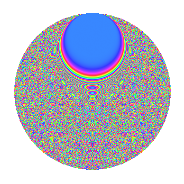# Properties

 Label 3024.2.heLevel 3024 Weight 2 Character orbit he Rep. character $$\chi_{3024}(155,\cdot)$$ Character field $$\Q(\zeta_{36})$$ Dimension 5184 Sturm bound 1152

# Related objects

## Defining parameters

 Level: $$N$$ = $$3024 = 2^{4} \cdot 3^{3} \cdot 7$$ Weight: $$k$$ = $$2$$ Character orbit: $$[\chi]$$ = 3024.he (of order $$36$$ and degree $$12$$) Character conductor: $$\operatorname{cond}(\chi)$$ = $$432$$ Character field: $$\Q(\zeta_{36})$$ Sturm bound: $$1152$$

## Dimensions

The following table gives the dimensions of various subspaces of $$M_{2}(3024, [\chi])$$.

Total New Old
Modular forms 6960 5184 1776
Cusp forms 6864 5184 1680
Eisenstein series 96 0 96

## Trace form

 $$5184q + O(q^{10})$$ $$5184q - 36q^{12} - 84q^{20} - 312q^{50} + 108q^{58} - 72q^{59} + 60q^{60} - 12q^{66} + 156q^{68} + 84q^{72} + 84q^{74} - 168q^{75} + 156q^{78} + 60q^{86} + 228q^{92} + O(q^{100})$$

## Decomposition of $$S_{2}^{\mathrm{new}}(3024, [\chi])$$ into newform subspaces

The newforms in this space have not yet been added to the LMFDB.

## Decomposition of $$S_{2}^{\mathrm{old}}(3024, [\chi])$$ into lower level spaces

$$S_{2}^{\mathrm{old}}(3024, [\chi]) \cong$$ $$S_{2}^{\mathrm{new}}(432, [\chi])$$$$^{\oplus 2}$$

## Hecke Characteristic Polynomials

There are no characteristic polynomials of Hecke operators in the database